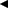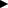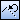# Dividing Shape

Divide a specified shape.
Shapes that can be divided are lines, polylines, circles, arcs, ellipses, elliptical arcs and B-Splines.
The following 4 methods can be used to divide shapes.

• Specifying dividing point.
• Specifying divided number.
• Specifying dividing length.
• Specifying dividing angle.

To display a command parameter to be executed, click/of command parameters, or press Tab key or / key on the keyboard.

1. Click [Divide] on  menu.
Then you will be able to divide a shape.
2.  Supplemental ▪ You can enter into the shape dividing status by clickingon  toolbar.
3. Click a shape to divide.

### When specifying dividing point

1. Specify a dividing point.
You can specify multiple points. Press Enter key on the keyboard, or clickon the toolbar on Command Window. Specifying point arrays will be complete.
2.  Supplemental ▪ To delete the last input point array, clickon the toolbar on Command Window.

### When specifying divided number

1. Input a divided number in [Divided Number] box on Command Window.
Shapes will be equally divided into the divided number.

### When specifying dividing length

1. Input a dividing length in [Dividing Length] box on Command Window.
Shapes will be divided with the specified length.
If a shape selected in Step 2 is other than a circle and ellipse, the endpoint nearer to the clicked position in the shape will be the start point of dividing.

### When specifying divide angle (when dividing circles, arcs, ellipses or elliptical arcs)

1. Input a dividing angle in [Dividing Angle] box on Command Window.
Shapes will be divided with the specified angle.
1. If a shape selected in Step 2 is other than a circle and ellipse, specify the start point of dividing.
When specifying start angle for dividing
Switch the parameter on Command Window to [Start Angle] box, and input the start angle of dividing.
When specifying start point for dividing
Switch the parameter on Command Window to [Start Point] box, and click the start point of dividing on the drawing.
 Supplemental ▪ You can specify a point array by inputting X and Y coordinates in [Dividing Point] and [Start Point] boxes on Command Window.